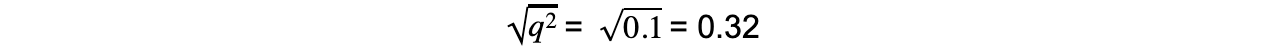# AQA A Level Biology复习笔记7.2.3 Hardy-Weinberg

### Natural Selection: Hardy-Weinberg Principle

• The Hardy-Weinberg principle states that if certain conditions are met then the allele frequencies of a gene within a population will not change from one generation to the next
• There are seven conditions or assumptions that must be met for the Hardy-Weinberg principle to hold true
• The Hardy-Weinberg equation allows for the calculation of allele and genotype frequencies within populations
• It also allows for predictions to be made about how these frequencies will change in future generations

#### Conditions for the Hardy-Weinberg principle

• For the Hardy-Weinberg principle to be correctly applied to a population a series of conditions, or assumptions, need to be met
• Organisms are diploid
• Organisms reproduce by sexual reproduction only
• There is no overlap between generations, i.e. parents do not mate with offspring
• Mating is random
• The population is large
• There is no migration, mutation, or selection
• This would mean no individuals entering the population (immigration) or leaving (emigration)
• Selection refers to both natural and artificial selection
• Allele frequencies are equal in both sexes
• The Hardy-Weinberg principle can be useful when building models and making predictions, but the assumptions listed are very rarely, if ever, all present in nature

#### Hardy-Weinberg equations

• If the phenotype of a trait in a population is determined by a single gene with only two alleles (we will use B / b as examples throughout this section) then the population will consist of individuals with three possible genotypes:
• Homozygous dominant (BB)
• Heterozygous (Bb)
• Homozygous recessive (bb)
•  When using the Hardy-Weinberg equation frequencies are represented as proportions of the population; a proportion is a number out of 1
• For example if every individual in the population has the homozygous dominant genotype BB then its frequency will be 1, while if half of the population show this genotype then the frequency will be 0.5
• Frequency of alleles can be represented; this is the proportion of all of the alleles in a population that are of a particular form
• The letter p represents the frequency of the dominant allele (B)
• The letter q represents the frequency of the recessive allele (b)
• As there are only two alleles at a single gene locus for a phenotypic trait in the population:

p + q = 1

• E.g. in a population of 100 individuals there would be 200 alleles because every individual has two versions of each gene
• If 120 of those alleles were the dominant allele then the frequency of the dominant allele would be 120/200
• It could be said that p = 120 ÷ 200 = 0.6
• If p = 0.6 then q = 1 - 0.6 = 0.4
• Frequency of genotypes can also be represented; this is the proportion of all of the individuals with a particular genotype
• The chance of an individual being homozygous dominant is p2
• The offspring would inherit dominant alleles from both parents so p x p = p2
•  The chance of an individual being heterozygous is 2pq
• Offspring could inherit a dominant allele from the father and a recessive allele from the mother (p x q) or offspring could inherit a dominant allele from the mother and a recessive allele from the father (p x q) so 2pq
• The chance of an individual being homozygous recessive is q2
•  The offspring would inherit recessive alleles from both parents so q x q = q2
•  As these are all the possible genotypes of individuals in the population the following equation can be constructed:

p2 + q2 + 2pq = 1

#### Worked Example

In a population of birds 10% of the individuals exhibit the recessive phenotype of white feathers. Calculate the frequencies of all genotypes.

Solution:

• We will use F / f to represent dominant and recessive alleles for feather colour
• Those with the recessive phenotype must have the homozygous recessive genotype, ff
• Therefore q2 = 0.10 (as 10% of the individuals have the recessive phenotype and q2 represents this)

To calculate the frequencies of the homozygous dominant ( p2 ) and heterozygous ( 2pq ):

Step 1: Find qStep 2: Find p (the frequency of the dominant allele F). If q = 0.32, and p + q = 1

p + q = 1

p = 1 - 0.32

p = 0.68

Step 3: Find p2 (the frequency of homozygous dominant genotype)

0.682 = 0.46

p2 = 0.46

Step 4: Find 2pq = 2 x (p) x (q)

2 x (0.68) x (0.32) = 0.44

Step 5: Check calculations by substituting the values for the three frequencies into the equation; they should add up to 1

p2 + 2pq + q2 = 1

0.46 + 0.44 + 0.10 = 1

In summary:

• Allele frequencies:
• p = F = 0.68
• q = f = 0.32

• Genotype frequencies:
• p2 = FF = 0.46
• q2 = ff = 0.10
• 2pq = Ff = 0.44

#### Exam Tip

When you are using Hardy-Weinberg equations you must always start your calculations by determining the proportion of individuals that display the recessive phenotype; this is the only phenotype from which you can immediately work out its genotype as it will always be homozygous recessive (the dominant phenotype is seen in both homozygous dominant and heterozygous individuals).

In Hardy-Weinberg questions it is a good idea to begin by establishing what information you have been given in the question (i.e. do you know q2, or do you know p?), and then establishing what the question wants you to work out (i.e. are you calculating 2pq?). You can then work out how to get from one to the other.

Don’t mix up the Hardy-Weinberg equations with the Hardy-Weinberg principle. The equations are used to estimate the allele and genotype frequencies in a population. The principle suggests that there is an equilibrium between allele frequencies and that there is no change in this between generations.